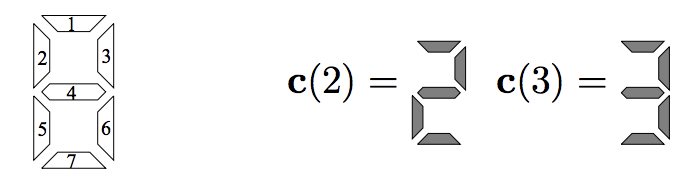# Neural Networks - Learning as Inference

## Motivation for the logistic function

The logistic function appears in problems where there is a binary decision to make. Here you will workout a problem (based on MacKays’s exercise 39.5) that like a binary neuron, also uses a logistic function.

### The noisy LED displayFigure 1. The noisy LED. Figure extracted from MacKay's Chapter 39.

In a LED display each number corresponds to a pattern of on(1) or off(0) for the 7 different elements that compose the display. For instance, the patterns for numbers 2 and 3 are:

$\mathbf{c}(2) = (1,0,1,1,1,0,1)$ $\mathbf{c}(3) = (1,0,1,1,0,1,1)$

Imagine you have a LED display that is not working properly. This defective LED is such that, for a given number the LED wants to display:

• Elements that have to be off, are wrongly on with probability $$f$$.

• Elements that have to be on, are actually on with probability $$1-f$$,

The LED is allowed to display ONLY a number “2” or a number “3”. And it does so by emitting a patter $$\mathbf{p}=(p1,p2,p3,p4,p5,p6,p7)$$, where $$p_i = 1,0$$

Calculate the posterior probability that the intended number was a “2”, given the pattern $$\mathbf{p}$$ you observe in the LED, that is,

$P(n=2\mid \mathbf{p}).$

Show that you can express that posterior probability as a logistic function,

$P(n=2\mid \mathbf{p}) = \frac{1}{1+e^{-\mathbf{w}\mathbf{p} + \theta}}$

for some weights $$\mathbf{w}$$, and some constant $$\theta$$.

You can assume that the prior probabilities for either number, $$P_2$$ and $$P_3$$, are given.

Hint: $$x^y = e^{y\log x}$$ for any two real numbers $$x, y$$.

### Solution

The probability that we can calculate is $$P(\mathbf{p}\mid 2)$$, that is the probability that observing a particular pattern $$\mathbf{p}$$, given that the LED tried to emit a “2”,

$P(\mathbf{p}\mid 2) = (1-f)^{p_1+p_3+p_4+p_5+p_7}\, f^{p2+p_6}.$

Using vector notation,

$P(\mathbf{p}\mid 2) = (1-f)^{\mathbf{c}(2)\mathbf{p}}\, f^{\mathbf{\hat c} (2)\mathbf{p}},$

where

${\hat c}(2)_i = 1- c(2)_i.$

Then using the hint above, we can rewrite,

\begin{aligned} P(\mathbf{p}\mid 2) &= e^{\log(1-f)\mathbf{c}(2)\,\mathbf{p}}\, e^{\log(f)\,\mathbf{\hat c} (2)\mathbf{p}}\\ &= e^{\log(1-f)\,\mathbf{c}(2)\mathbf{p} + \log(f)\,\mathbf{\hat c} (2)\mathbf{p}}\\ &= e^{\left[\log(1-f)\,\mathbf{c}(2) + \log(f)\,\mathbf{\hat c} (2)\right]\mathbf{p}}.\\ \end{aligned}

Introducing the vector

$\mathbf{a}(2) = \log(1-f)\,\mathbf{c}(2) + \log(f)\,\mathbf{\hat c} (2)$

such that

$a(2)_i = \log(1-f)\,c(2)_i + \log(f)\,\left(1-c(2)_i\right),$

we can write with all generality

$P(\mathbf{p}\mid 2) = e^{\mathbf{a}(2)\,\mathbf{p}}.$

The quantity we have been asked to calculate is not $$P(\mathbf{p}\mid 2)$$, but instead, given that we have seen a pattern $$\mathbf{p}$$, what is the probability that the pattern was generated with a “2” in mind. That is the posterior probability $$P(2\mid \mathbf{p})$$, which using Bayes theorem is given as a function of $$P(\mathbf{p}\mid 2)$$ as

$P(2\mid \mathbf{p}) = \frac{P(\mathbf{p}\mid 2) P(2)}{P(\mathbf{p})},$

where $$P(2)$$ is a prior probability.

In the general case in which the LED can produce any of the 10 digits (from 0 to 9), then we have by marginalization

\begin{aligned} P(\mathbf{p}) &= P(\mathbf{p}\mid 0) P(0) + P(\mathbf{p}\mid 1) P(1) + P(\mathbf{p}\mid 2) P(2) + \ldots + P(\mathbf{p}\mid 9) P(9)\\ &= \sum_{n=0}^{9} e^{\mathbf{a}(n)\,\mathbf{p}}\, P(n). \end{aligned}

Resulting in the general solution,

$P(2\mid \mathbf{p}) = \frac{e^{\mathbf{a}(2)\,\mathbf{p}}\, P(2)}{\sum_{n=0}^{9} e^{\mathbf{a}(n)\,\mathbf{p}}\, P(n)},$

Notice that, the normalization condition is $$\sum_{n=0}^9 P(n\mid \mathbf{p}) = 1$$.

For our particular problem, where we want to distinguish only between the pattern being generated by a “2” or a “3”, that results in

$P(2\mid \mathbf{p}) = \frac{e^{\mathbf{a}(2)\,\mathbf{p}}\, P(2)}{e^{\mathbf{a}(2)\,\mathbf{p}}\, P(2) + e^{\mathbf{a}(3)\,\mathbf{p}}\, P(3)},$

where here the normalization condition is

$P(2\mid \mathbf{p}) + P(3\mid \mathbf{p}) = 1.$

The posterior probability $$P(2\mid \mathbf{p})$$ can be re-written as

$P(2\mid \mathbf{p}) = \frac{1}{1 + e^{\left[\mathbf{a}(3)-\mathbf{a}(2)\right]\,\mathbf{p}}\, \frac{P(3)}{P(2)}}.$

We can define the weights

$\mathbf{w} = \mathbf{a}(3)-\mathbf{a}(2).$

We can also parameterize the priors as

$\frac{P(3)}{P(2)}=e^\theta.$

For instance, $$\theta = -\log(2)$$ for $$P(2) = P(3) = 1/2$$.

Then, we have the expression we wanted to obtain of $$P(2\mid \mathbf{p})$$ as a logistic linear function

$P(2\mid \mathbf{p}) = \frac{1}{1 + e^{\mathbf{w}\,\mathbf{p} + \theta}},$

with weights,

\begin{aligned} w_i &= a(3)_i - a(2)_i\\ &= \log(1-f)\,\left[c(3)_i - c(2)_i\right] + \log(f)\,\left[c(2)_i - c(3)_i\right]\\ &=\frac{\log(1-f)}{\log f}\left[c(3)_i-c(2)_i\right]\\ \end{aligned}

or

$\mathbf{w} = \frac{\log(1-f)}{\log f}\left(0, 0, 0, 0, -1, 1, 0\right).$